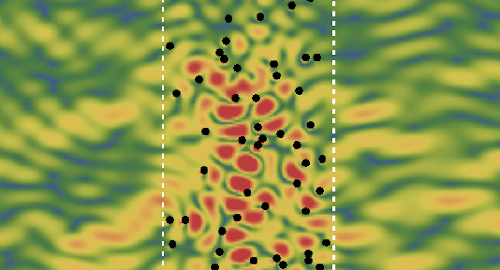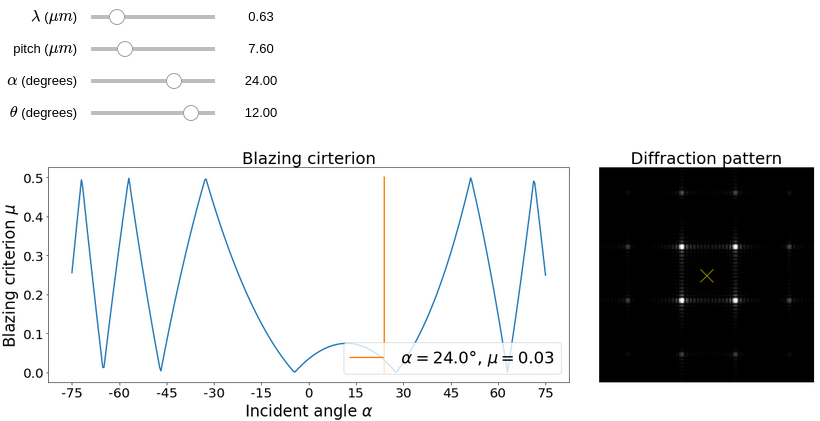Created by sebastien.popoff on 05/11/2020

## Fast numerical estimations of axisymmetric multimode fibers modesEstimating the propagation constants and the transverse mode profiles of multimode fibers is not as easy as it sounds. In our recent work we highlighted here, we needed to estimate the mode profiles for a standard graded-index fiber. It turned out that many standard approximations done in the literature to estimate the propagation constants do not give results accurate enough for the mode profile. The general approach we introduced in a previous tutorial to numerically find the fiber modes for any index profile using a 2D scalar finite differences approach is still valid. However, to provide accurate results, it needs a fine discretization of the space that leads to important memory and computational time requirements when the fiber core increases. If we consider an axisymmetric fiber, we can obtain a 1D formulation of the problem, that is unfortunately unstable under naive finite differences approaches. We detail here a stable formulation that leads to accurate and fast estimations of the mode profiles.

Created by sebastien.popoff on 16/09/2020

## Langevin Institute, ParisWe propose a 2 years postdoctoral position in the Waves Theory and Mesoscopic Physics group of the Langevin Institute under the supervision of Arthur Goetschy. The goal of the project is to provide a theory for the scattering matrix of strongly scattering media made of resonant units for wavefront shaping applications. Applicants should have a Ph.D. in wave physics with a solid background on wave propagation in complex systems.
Contact: arthur.goetschy@espci.psl.eu

Created by sebastien.popoff on 23/06/2020

## DMD diffraction toolI presented in this tutorial the diffraction effects occurring in a DMD setup. It corresponds to a blazed grating effect and depends on the wavelength $$\lambda$$, the pixel pitch $$d$$, the incident angle $$\alpha$$, and the angle of the micro-mirrors $$\theta$$. Here is a simple app (see source code on Github) to calculate the criterion for optimal diffraction efficiency and the aspect of the diffraction pattern in the far-field when illuminating the DMD with a plane wave of incident angle $$\alpha$$ with respect to the normal of the surface.

Created by sebastien.popoff on 22/05/2019

## Semidefinite Programming for Intensity Only Estimation of the Transmission Matrix

The possibility of measuring the transmission matrix using intensity only measurements is a much sought-after feature as it allows us not to rely on interferometry. Interferometry usually requires laboratory-grade stability difficult to obtain for real-world applications. Typically, we want to be able to retrieve the transmission matrix from a set of pairs composed of input masks and output intensity patterns. However, this problem, which corresponds to a phase-retrieval problem, is not convex, hence difficult to solve using standard techniques. The idea proposed in [I. Waldspurger et al., Math. Program (2015)] is to relax some constraints to approximate the problem to a convex one that can be solved using the semidefinite programming approach. I briefly detail the approach and provide an example of the procedure to reconstruct the transmission matrix using Python. A Jupyter notebook can be found on my Github account: semidefiniteTM_example.ipynb.